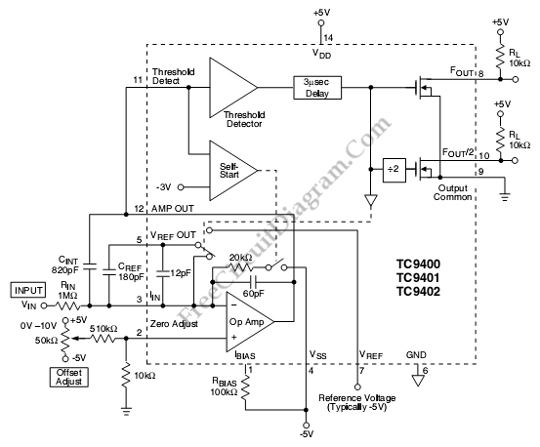# TC9400 10Hz to 10kHz Voltage-to-Frequency ConverterThis is a TC9400 10Hz to 10kHz Voltage-to-Frequency Converter circuit. This ciruit uses TC9400 to convert voltage to frequency. The TC9400 uses charge balancing principal. This circuit uses input resistor to convert the input voltage to input current. Then the capacitor will change the current into charge which will decrease the Op amp output voltage linearly. This circuit also featured by threshold detector which set the lower limit of output swing. That will make the capacitor charged to reference voltage. The output of op-amp is a finite amount because the charge on the integrating capacitor is reduced by fixed amount(q=Vref X Cref). Here is the schematic diagram of the circuit:The Creff will shorted out at the end of charging period. The system will ready to recycle, When the crosses zero. The integrating capacitor is discharged by input balance out by fixed charges from the reference voltage continuously. The number of reference pulses needed to maintain the balance will increase when the input voltage is increased. So the output frequency also increase. The increase in voltage and frequency is linear because each charge increment is fixed. This circuit has a good accuracy in the width of  output pulse, but it does not affect the linearity of the V/F directly. To take place, the pulse must long enough for full charge transfer. [Circuit’s schematic diagram source: Microchip Datasheet]